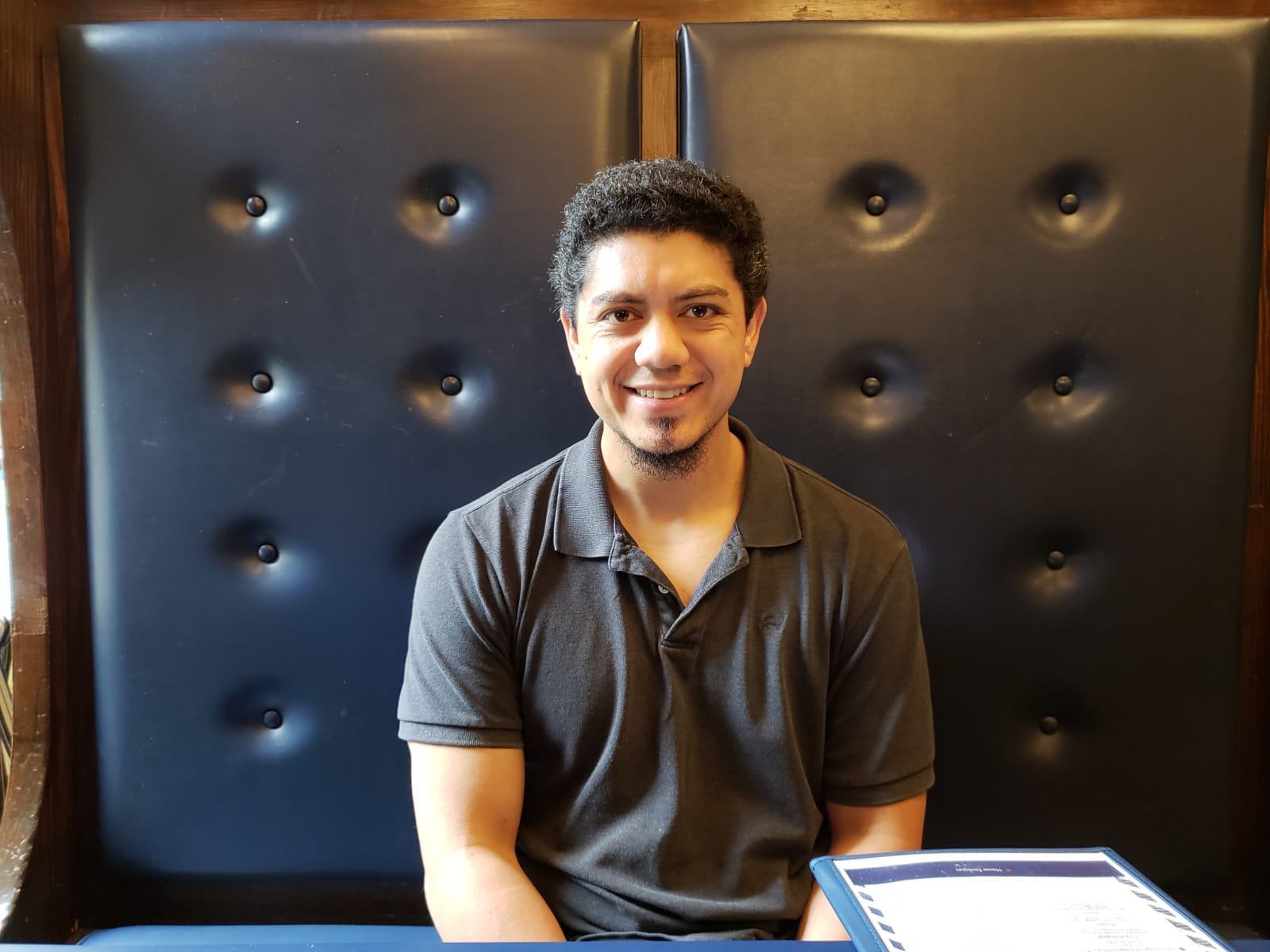# Web Page of Alejandro GinoryAlejandro Ginory
Department of Mathematics - Hill Center
Rutgers, The State University Of New Jersey
110 Frelinghuysen Rd.
Piscataway, NJ 08854-8019

### Blurb:

My interests include representation theory, algebraic combinatorics, and experimental mathematics. More importantly, I am currently thinking about Verlinde algebras, affine Kac-Moody Lie algebras, theta functions, non-homogeneous symmetric functions, convergence of iterates of a nonlinear operators, representations of double affine Hecke algebras, and undergraduate mathematical proof comprehension.

### Office:

Room 618, Hill Center, Busch Campus

Hours: 2 - 3 PM Tuesdays

Directions: There are two pairs of elevators in the Hill building. One of them goes up to the fifth floor and the other goes up to the seventh. Choose wisely.

aginory at math dot rutgers dot edu

### Random Math 351 Exercises:

• Prove that for every pair of integers a and b (not both zero), every common divisor of a and b divides the greatest common divisor of a and b.
• Let R be a ring with identity element 1 and let S be the set {0,1,2,...,n,n+1,...}. Prove that if R is an integral domain and S is finite, then |S| is prime.
• (a) Find examples of commutative rings R (not necessarily with identity) and maximal ideals m such that m is NOT a prime ideal.
(b) Let R be a commutative ring (not necessarily with identity) such that for every x in R, x is in Rx. Prove that all maximal ideals in R are prime ideals.
• Let p be a prime number. Prove: If G is a group of order p, then G is cyclic. Also prove: If G is a group of order p^2, then G is abelian.

### Preprints (and in preparation)

A. Ginory, J. Kim. Weingarten Calculus and the IntHaar Package for Integrals over Compact Matrix Groups
A. Ginory. Twisted Affine Lie Algebras, Fusion Algebras, and Congruence Subgroups
A. Ginory, R. Nussbaum. On the Border of Order-Preserving Maps: A Problem Suggested by Lie Algebra Theory
A. Ginory. Vertex Algebras Conformal Blocks and Zhu's algebra

### Maple Packages

IntHaar (accompanying paper "Weingarten Calculus and the IntHaar package for Integrals over Compact Matrix Groups" (on ArXiv)
Simple and Affine Lie Algebras - Computes Cartan matrices, Coxeter numbers, dual Coxeter numbers, and other related data for simple and affine Lie algebras.(in progress)
Lattice Program - Computes dual lattices, bases, sums, intersections and other linear algebra related calculations.(in progress)

### Old Seminars

Graduate Algebra and Representation Theory Seminar - Spring 2019
Graduate Algebra and Representation Theory Seminar - Fall 2018
Graduate Algebra and Representation Theory Seminar - Spring 2018
Graduate Algebra and Representation Theory Seminar - Fall 2017
Graduate Student Vertex Operator Algebra Seminar - Spring 2017
Graduate Student Vertex Operator Algebra Seminar - Fall 2016

Math

### Some Notes

My Rough Notes for Finite Group Representation Theory
Vertex Operator Algebra Notes (in progress, last update 10/27/16)
The Square Root of 2 is Irrational
The Brouwer Fixed Point Theorem
Counting Factorizations in the Symmetric Group
de Rham's Theorem
Closed Subgroup Theorem (Lie Groups)
Equivalence of 2 Classical Constructions of the p-adic Numbers

IntU stuff
Thesis Slides